Cody

# Problem 42477. Model a simple pendulum

Model a simple pendulum of length 200cm with bob of mass 100g and plot the position in degrees. The pendulum starts at 30 degrees from vertical position to the left as shown in the figure below: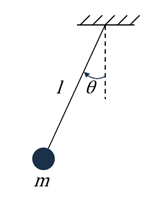The mass, length and initial position of the pendulum are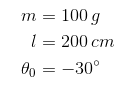The standard acceleration due to gravity is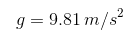and recall that the equations of motion are given as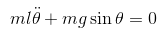### Solution Stats

36.38% Correct | 63.62% Incorrect
Last solution submitted on Mar 14, 2019

#### More from this Author14

You Can Use Simulink

#### Tags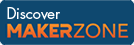MATLAB and Simulink resources for Arduino, LEGO, and Raspberry Pi